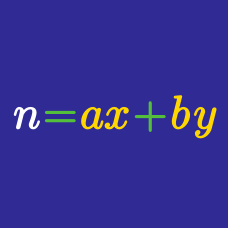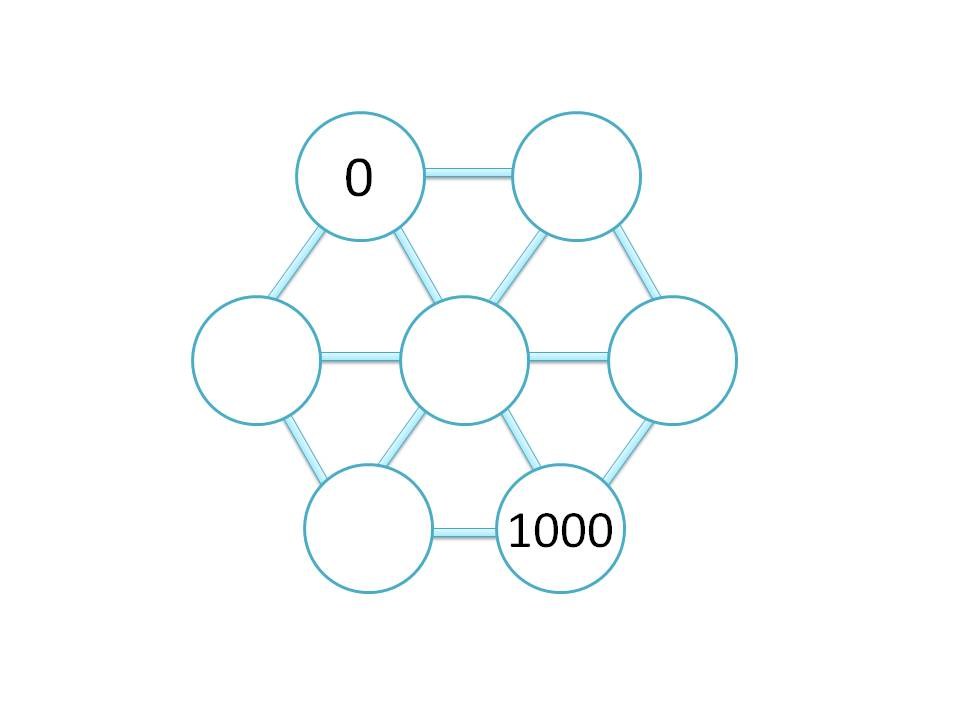Number Theory

# Linear Diophantine Equations: Level 3 Challenges

Solve the following cryptarithm:

$\begin{array} { l l l l l l } & & S & E & N & D \\ +& & M & O & R & E \\ \hline & M & O & N & E & Y \\ \end{array}$

and find the value of $$S+E+N+D+M+O+R+Y.$$

I am thinking of a four digit positive integer with distinct digits.

Of course, there's a total of $$4!-1=23$$ ways to rearrange the digits to form a new 4 digit positive integer.

If the sum of these other 23 numbers is 157193, what is the number that I was thinking of?

What is the smallest positive integer that can be written as the sum of 39 positive consecutive integers, the sum of 40 positive consecutive integers, and the sum of 41 positive consecutive integers?Each circle has three or six neighbors, and the number placed in each blank circle must be the average of its neighbors. What is the largest of the five missing numbers?

Find the number of ordered pairs of positive integer solutions $$(m, n)$$ for $20m + 12n = 2012.$

×